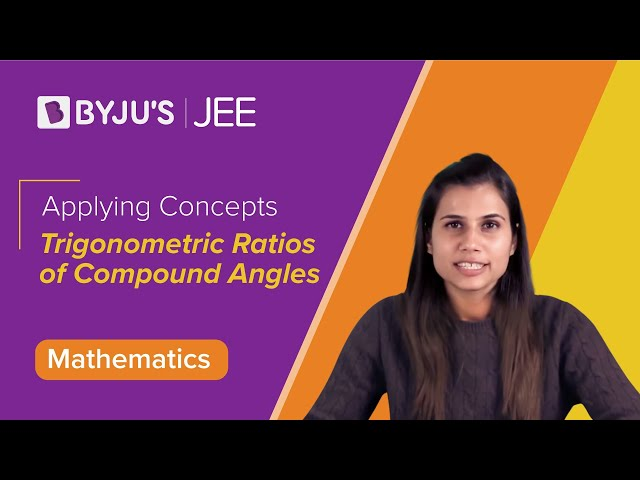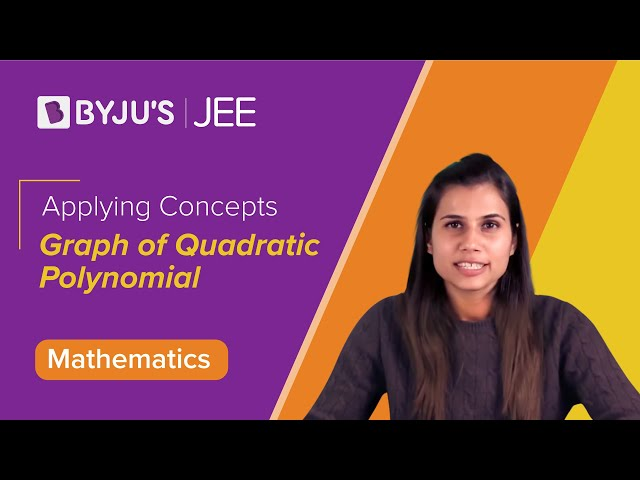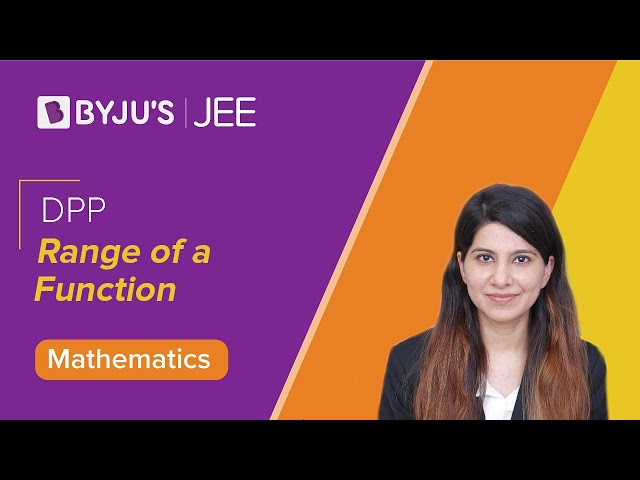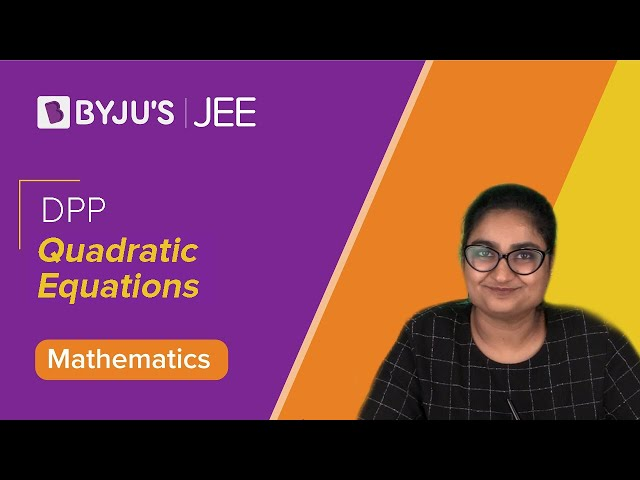Win up to 100% scholarship on Aakash BYJU'S JEE/NEET courses with ABNAT Win up to 100% scholarship on Aakash BYJU'S JEE/NEET courses with ABNAT

# JEE Main 2020 Maths Paper With Solutions Jan 7 Shift 1

JEE Main 2020 solved shift 1 Maths paper consists of step by step solutions, prepared by our subject experts. Students are recommended to practise these solutions so that they can learn the problems easily. These solutions help students to get an idea of what type of questions are asked for the JEE Main exam. Practising these solutions will definitely boost the confidence of the students. Students can easily access the solutions from our webpage.
January 7 Shift 1 – Maths
Question 1. The area of the region, enclosed by the circle x2 + y2 = 2 which is not common to the region bounded by the parabola y2 = 𝑥 and the straight line 𝑦 = 𝑥, is
a) (1/3)(12𝜋 − 1)
b) (1/6)(12𝜋 − 1)
c) (1/3)(6𝜋 − 1)
d) (1/6)(24𝜋 − 1)

Required area = area of the circle – area bounded by given line and parabola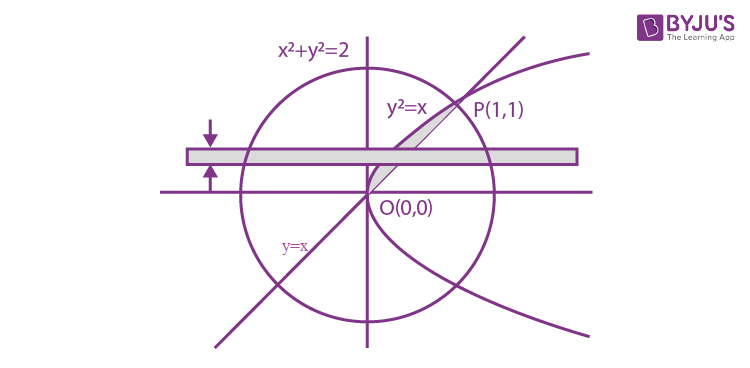Required area = πr2

$$\begin{array}{l}\int_{0}^{1}(y-y^{2})dy\end{array}$$

Area =

$$\begin{array}{l}2\pi -(\frac{y^{2}}{2}-\frac{y^{3}}{3})_{0}^{1}\end{array}$$

= 2𝜋 – (1/6)

= (1/6)(12𝜋 – 1)

Question 2. Total number of six-digit numbers in which only and all the five digits 1,3,5,7 and 9 appear, is
a) 56
b) (½)(6!)
c) 6!
d) (5/2) 6!

Selecting all 5 digits = 5C5 = 1 way

Now, we need to select one more digit to make it a 6 digit number = 5C1 = 5 ways

Total number of permutations =

$$\begin{array}{l}\frac{6!}{2!}\end{array}$$

Total numbers = 5C5×5C1×(6!/2!)

= (5/2) 6!

Question 3. An unbiased coin is tossed 5 times. Suppose that a variable 𝑋 is assigned the value 𝑘 when 𝑘 consecutive heads are obtained for 𝑘 = 3, 4, 5, otherwise 𝑋 takes the value -1. The expected value of 𝑋, is
a) 1/8
b) 3/16
c) -1/8
d) -3/16

k = no. of consecutive heads

P(k = 3) = 5/32 {HHHTH, HHHTT, THHHT, HTHHH, TTHHH}

P(k = 4) = 2/32 {HHHHT, THHHH}

P(k = 5) = 1/32 {HHHHH}

$$\begin{array}{l}P(\bar{3}\cap \bar{4}\cap \bar{5})= 1-(\frac{5}{32}+\frac{2}{32}+\frac{1}{32}) = \frac{24}{32}\end{array}$$

ƩXP(X) =

$$\begin{array}{l}(-1\times \frac{24}{32})+(3\times \frac{5}{32})+(4\times \frac{2}{32})+(5\times \frac{1}{32})\end{array}$$

= 1/8

Question 4. If Re (z-1)/(2z + i) = 1, where z = x+iy, then the point (x,y) lies on a
a) circle whose centre is at (-1/2, -3/2)
b) straight line whose slope is 3/2.
c) circle whose diameter is √5/2
d) straight line whose slope is -2/3.

z = x + iy

$$\begin{array}{l}\frac{x+iy-1}{2x+2iy+i} = \frac{(x-1)+iy}{2x+i(2y+1)}\left ( \frac{2x-i(2y+1)}{2x-i(2y+1)}\right )\end{array}$$
$$\begin{array}{l}\frac{2x(x-1)+y(2y+1)}{4x^{2}+(2y+1)^{2}}= 1\end{array}$$

2x2 + 2y2 – 2x + y = 4x2 + 4y2 + 4y + 1

x2 + y2 + x + (3/2)y + (1/2) = 0

Circle’s centre will be (-1/2, -3/4)

Radius = √[(1/4) + (9/16) – (1/2)] = √5/4

Diameter = √5/2

Question 5. If f(a + b + 1 – x) = f(x) ∀x, where a and b are fixed positive real numbers, then
$$\begin{array}{l}\frac{1}{(a+b)}\int_{a}^{b}x(f(x)+f(x+1))dx\end{array}$$
is equal to

a)
$$\begin{array}{l}\int_{a-1}^{b-1}f(x)dx\end{array}$$

b)
$$\begin{array}{l}\int_{a+1}^{b+1}f(x+1)dx\end{array}$$

c)
$$\begin{array}{l}\int_{a-1}^{b-1}f(x+1)dx\end{array}$$

d)
$$\begin{array}{l}\int_{a+1}^{b+1}f(x)dx\end{array}$$

f(a + b + 1 – x) = f(x) …(i)

f(a + b – x) = f(x+1) …(ii)

$$\begin{array}{l}I = \frac{1}{(a+b)}\int_{a}^{b}x(f(x)+f(x+1))dx\end{array}$$
…(iii)

From (i) and (ii)

$$\begin{array}{l}I = \frac{1}{(a+b)}\int_{a}^{b}(a+b-x)(f(x+1)+f(x))dx\end{array}$$
…(iv)

$$\begin{array}{l}2I = \int_{a}^{b}(f(x)+f(x+1))dx\end{array}$$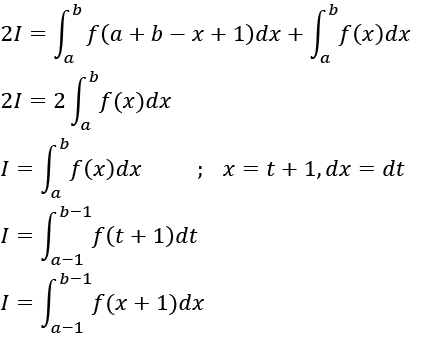Question 6. If the distance between the foci of an ellipse is 6 and the distance between its directrices is 12, then the length of its latus rectum is
a) 2√3
b) √3
c) 3/√2
d) 3√2

Let the equation of ellipse be

x2/a2 + y2/b2 = 1

a>b

Now 2ae = 6

2a/e = 12

ae = 3 and a/e = 6

a2 = 18

a2e2 = c2 = a2-b2 = 9

b2 = 9

length of latus rectum = 2b2/a = 2×9/√18 = 3√2

Question 7. The logical statement (p⇒q) ∧ (q ⇒∼ p) is equivalent to
a) ∼ p
b) p
c) q
d) ∼ q

 𝒑 𝒒 𝒑 ⇒ 𝒒 ∼ 𝒑 𝒒 ⇒∼ 𝒑 (𝒑 ⇒ 𝒒) ∧ (𝒒 ⇒∼ 𝒑) T T T F F F T F F F T F F T T T T T F F T T T T

Clearly (𝑝 ⇒ 𝑞) ∧ (𝑞 ⇒∼ 𝑝) is equivalent to ∼ 𝑝.

Question 8. The greatest positive integer 𝑘, for which 49𝑘 + 1 is a factor of the sum 49125 + 49124 + ⋯ + 492 + 49 + 1, is
a) 32
b) 60
c) 65
d) 63

1 + 49 + 492 + …. + 49125 = (49126 – 1)/(49 – 1)

= (4963 + 1)( 4963 – 1)/48

= (4963 + 1)((1 + 48)63-1)/48

= (4963 + 1)(1 +48)63 -1)/48

= (4963 + 1)(1 +48 I-1)/48: where I is an integer

= (4963 + 1)I

Greatest positive integer is k = 63

Question 9. A vector 𝑎⃗ = 𝛼𝑖̂ + 2𝑗̂ + 𝛽𝑘̂ (𝛼, 𝛽 ∈ 𝑹) lies in the plane of the vectors, ⃗𝑏 = 𝑖̂ + 𝑗̂ and 𝑐⃗ = 𝑖̂ − 𝑗̂ + 4𝑘̂. If 𝑎⃗ bisects the angle between 𝑏⃗ and 𝑐⃗, then
a) 𝑎⃗. 𝑖̂ + 3 = 0
b) 𝑎⃗. 𝑘̂ + 4 = 0
c) 𝑎⃗. 𝑖̂ + 1 = 0
d) 𝑎⃗. 𝑘̂ + 2 = 0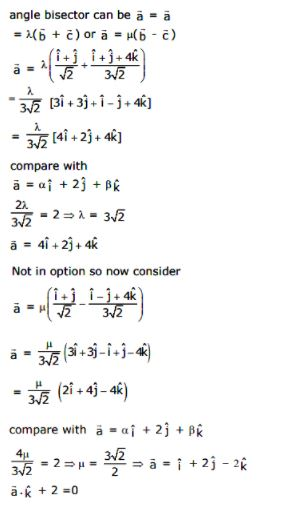Question 10. If y(α) =
$$\begin{array}{l}\sqrt{2(\frac{\tan \alpha + \cot \alpha }{1+\tan ^{2}\alpha })+\frac{1}{\sin ^{2}\alpha }}\end{array}$$
where α ∈ (3π/4, π) then dy/dα at α = 5π/6 is

a) -1/4
b) 4/3
c) 4
d) -4

y(α) =

$$\begin{array}{l}\sqrt{2(\frac{\tan \alpha + \cot \alpha }{1+\tan ^{2}\alpha })+\frac{1}{\sin ^{2}\alpha }}\end{array}$$
$$\begin{array}{l}y(\alpha )= \sqrt{2\frac{1}{\sin \alpha \cos \alpha \times \frac{1}{\cos ^{2}\alpha }}+\frac{1}{\sin ^{2}\alpha }}\end{array}$$
$$\begin{array}{l}y(\alpha )= \sqrt{2\cot \alpha +cosec^{2} \alpha }\end{array}$$

y(α) = √(1+cotα )2

y(α) = -1 – cotα

dy/dα = 0 + cosec2α

dy/dα = cosec2 5π/6

dy/dα = 4

Question 11. If y = mx + 4 is a tangent to both the parabolas, y2 = 4x and x2 = 2by, then b is equal to
a) -64
b) 128
c) -128
d) -32

Any tangent to the parabola y2 = 4x is y = mx + a/m

Comparing it with y = mx + 4, we get 1/m = 4

So m = ¼.

Equation of tangent becomes y = (x/4) + 4

y = (x/4) + 4 is a tangent to x2 = 2by

x2 = 2b{(x/4)+4}

Or 2x2 – bx – 16b = 0

D = 0

b2 + 128b = 0

b = 0 (not possible)

b = -128

Question 12. Let 𝛼 be a root of the equation x2 + x+ 1= 0 and the matrix A =
$$\begin{array}{l}\frac{1}{\sqrt{3}}\begin{bmatrix} 1 & 1 & 1\\ 1& \alpha & \alpha ^{2}\\ 1& \alpha ^{2} & \alpha ^{4} \end{bmatrix}\end{array}$$
then the matrix A31 is equal to

a) A
b) A2
c) A3
d) I3

The roots of equation 𝑥2 + 𝑥 + 1=0 are complex cube roots of unity.

= ω or ω2

$$\begin{array}{l}A= \frac{1}{\sqrt{3}}\begin{bmatrix} 1 & 1 & 1\\ 1& \alpha & \alpha ^{2}\\ 1& \alpha ^{2} & \alpha ^{4} \end{bmatrix}\end{array}$$

=

$$\begin{array}{l}\frac{1}{\sqrt{3}}\begin{bmatrix} 1 & 1 & 1\\ 1& \omega & \omega ^{2}\\ 1& \omega ^{2} & \omega ^{4} \end{bmatrix}\end{array}$$

A2 =

$$\begin{array}{l}\frac{1}{{3}}\begin{bmatrix} 1 & 1 & 1\\ 1& \omega & \omega ^{2}\\ 1& \omega ^{2} & \omega ^{4} \end{bmatrix}\begin{bmatrix} 1 & 1 & 1\\ 1& \omega & \omega ^{2}\\ 1& \omega ^{2} & \omega ^{4} \end{bmatrix}\end{array}$$
$$\begin{array}{l}A^{2}=\frac{1}{3}\begin{bmatrix} 3 & 0 & 0\\ 0 & 0 & 3\\ 0 & 3 & 0 \end{bmatrix}\end{array}$$
$$\begin{array}{l}A^{2}=\begin{bmatrix} 1 & 0 & 0\\ 0 & 0 & 1\\ 0 & 1 & 0 \end{bmatrix}\end{array}$$
$$\begin{array}{l}A^{4}=\begin{bmatrix} 1 & 0 & 0\\ 0 & 0 & 1\\ 0 & 1 & 0 \end{bmatrix}\begin{bmatrix} 1 & 0 & 0\\ 0 & 0 & 1\\ 0 & 1 & 0 \end{bmatrix}\end{array}$$
$$\begin{array}{l}A^{4}=\begin{bmatrix} 1 & 0 & 0\\ 0 & 0 & 1\\ 0 & 1 & 0 \end{bmatrix}= I\end{array}$$

A4 = I

A28 = 𝐼

Therefore, we get

A31 = A28 A3

A31 = IA3

A31 = A3

Question 13. If g(x) = x2 + x – 1 and (gof)(x) = 4x2 – 10x + 5, then f(5/4) is equal to
a) -3/2
b) -1/2
c) 1/2
d) 3/2

g(x) = x2 + x – 1

gof(x) = 4x2 – 10x + 5

g(f(x) = 4x2 – 10x + 5

f2(x) + f(x) – 1 = 4x2 – 10x + 5

Putting x = 5/4 and f(5/4) = t

t2 + t + ¼ = 0

t = -1/2 or f(5/4) = -1/2

Question 14. Let 𝛼 and 𝛽 are two real roots of the equation (𝑘 + 1) tan2 𝑥 − √2 𝜆 tan 𝑥 = 1 − 𝑘, where (𝑘 ≠ −1) and are real numbers. If tan2(α + β) = 50, then value of 𝜆 is
a) 5√2
b) 10√2
c) 10
d) 5

(k+1) tan2x – √2 tan x = 1 – k

tan2(α + β) = 50

∵ tan α and tan β are the roots of the given equation

Now

tan α + tanβ = √2/(k+1),

tanα tan β= (k -1)/(k+1)

$$\begin{array}{l}\left ( \frac{\frac{\sqrt{2 }\lambda}{k+1}}{1-\frac{k-1}{k+1}} \right )^{2} = 50\end{array}$$

2/4 = 50

λ2 = 100

λ = 10, λ = -10

Question 15. Let P be a plane passing through the points (2, 1, 0), (4, 1, 1) and (5, 0, 1) and 𝑅 be any point (2, 1, 6). Then the image of 𝑅 in the plane 𝑃 is:
a) (6, 5, 2)
b) (6, 5, −2)
c) (4,3, 2)
d) (3,4, −2)

Points A(2, 1, 0), B(4, 1, 1) C(5, 0, 1)

$$\begin{array}{l}\vec{AB } = (2,0,1)\end{array}$$
$$\begin{array}{l}\vec{AC } = (3,-1,1)\end{array}$$
$$\begin{array}{l}\vec{n } = \vec{AB}\times \vec{AC }\end{array}$$

Equation of the plane is x + y -2z = 3….(1)

Let the image of point (2, 1, 6) is (l, m, n)

(l-2)/1 = (m-1)/1 = (n-6)/-2 = -2(-12)/6 = 4

⇒ l = 6, m = 5, n = −2

Hence the image of R in the plane P is (6, 5, −2)

Question 16. Let xk + yk = ak, (a,k>0) and (dy/dx) + (y/x)1/3 = 0, then k is
a) 1/3
b) 3/2
c) 2/3
d) 4/3

xk + yk = ak

kxk-1 + kyk-1 (dy/dx) = 0

dy/dx = -(x/y)k-1

dy/dx + (y/x)1-k = 0

1-k = 1/3

k = 2/3

Question 17. Let the function, f:[-7, 0] →R be continuous on [−7, 0] and differentiable on (−7, 0). If f(-7)= −3 and 𝑓(𝑥) ≤ 2, for all 𝑥 ∈ (−7, 0), then for all such functions 𝑓, 𝑓(−1) + 𝑓(0) lies in the interval:
a) [-6, 20]
b) (-∞, 20]
c) (-∞, 11]
d) [-3, 11]

f(-7) = -3 and f’(x) ≤ 2

Applying LMVT in [-7,0], we get

(f(-7) – f(0))/-7 = f’(c) ≤ 2

(-3-f(0))/-7 ≤ 2

f(0) + 3 ≤ 14

f(0) ≤ 11

Applying LMVT in [-7, -1], we get

(f(-7) – f(-1))/(-7 +1) = f’(c) ≤ 2

-3 – f(-1))/-6 = f’(c) ≤ 2

f(-1) + 3 = ≤ 12

f(-1) ≤ 9

Therefore f(-1)+ f(0) ≤ 20

Question 18. If y = y(x) is the solution of the differential equation ey(dy/dx)-1 = ex such that y(0) = 0, then y(1) is equal to
a) loge 2
b) 2e
c) 2 + loge 2
d) 1+loge 2

𝑒y(𝑦 − 1) = 𝑒𝑥

⇒ dy/dx = 𝑒𝑥−𝑦 + 1

Let x-y = t

1 – dy/dx = dt/dx

So, we can write

⇒ 1 − dt/dx = 𝑒𝑡 + 1

⇒ −𝑒−𝑡 𝑑𝑡 = 𝑑𝑥

⇒ 𝑒−𝑡 = 𝑥 + 𝑐

⇒ 𝑒𝑦−𝑥 = 𝑥 + 𝑐

1 = 0 + 𝑐

⇒ 𝑒𝑦−𝑥 = 𝑥 + 1

at 𝑥 = 1

⇒ 𝑒𝑦−1 = 2

⇒ 𝑦 = 1 + log22

Question 19. Five numbers are in A.P., whose sum is 25 and product is 2520. If one of these five numbers is -1/2, then the greatest number amongst them is
a) 16
b) 27
c) 7
d) 21/2

Let 5 numbers be 𝑎 − 2𝑑, 𝑎 − 𝑑, 𝑎, 𝑎 + 𝑑, 𝑎 + 2𝑑

5𝑎 = 25

𝑎 = 5

(𝑎 − 2𝑑)(𝑎 − 𝑑) a (𝑎 + 𝑑)(𝑎 + 2𝑑) = 2520

(25 − 4𝑑2)(25 − 𝑑2) = 504

4𝑑4 − 125𝑑2 + 121 = 0

4𝑑4 − 4𝑑2 − 121𝑑2 + 121 = 0

𝑑2 = 1 𝑜𝑟 𝑑2 = 121/4

d = 11/2

For d = 11/2, a + 2d is the greatest term, a + 2d = 5 + 11 = 16.

Question 20. If the system of linear equations

2x + 2ay + az = 0

2x + 3by + bz = 0

2x + 4cy + cz = 0,

where a, b, c ∈ R are non-zero and distinct; has non-zero solution, then

a) a + b + c = 0
b) a, b, c are in A.P
c) 1/a, 1/b, 1/c are in A.P
d) a, b, c are in G.P

$$\begin{array}{l}\begin{vmatrix} 2 & 2a &a \\ 2& 3b &b \\ 2& 4c & c \end{vmatrix}=0\end{array}$$

R2 R2 – R1

R3 R3 – R1

$$\begin{array}{l}\begin{vmatrix} 2 & 2a &a \\ 0& 3b-2a &b-a \\ 0& 4c-2a & c-a \end{vmatrix}=0\end{array}$$

⇒ (3b − 2𝑎)(𝑐 − 𝑎) − (4𝑐 − 2𝑎)(𝑏 − 𝑎) = 0

⇒ 3𝑏𝑐 − 2𝑎𝑐 − 3𝑎𝑏 + 2𝑎2 − [4𝑏𝑐 − 4𝑎𝑐 − 2𝑎𝑏 + 2𝑎2] = 0

⇒ −𝑏𝑐 + 2𝑎𝑐 − 𝑎𝑏 = 0

⇒ 𝑎𝑏 + 𝑏𝑐 = 2𝑎𝑐

1/c + 1/a = 2/b

Question 21.
$$\begin{array}{l}\lim_{x\to 2}\frac{3^{x}+3^{3-x}-12}{3^{-\frac{x}{2}}-3^{1-x}}\end{array}$$
is equal to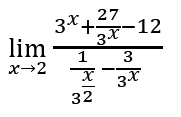Put 3x/2 = t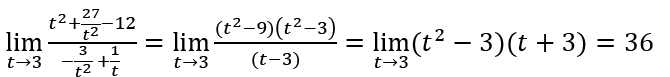Question 22.If variance of first 𝑛 natural numbers is 10 and variance of first 𝑚 even natural numbers is 16, 𝑚 + 𝑛 is equal to

For 𝑛 natural number variance is given by

$$\begin{array}{l}\sigma ^{2} = \frac{\sum x_{i}^{2}}{n}- (\frac{\sum x_{i}}{n})^{2}\end{array}$$
$$\begin{array}{l}\frac{\sum x_{i}^{2}}{n}= \frac{1^{2}+2^{2}+..n^{2}}{n} = \frac{n(n+1)(2n+1)}{6n}\end{array}$$
$$\begin{array}{l}\frac{\sum x_{i}}{n}= \frac{1+2+..n}{n} = \frac{n(n+1)}{2n}\end{array}$$

σ2 = (n2-1)/12 = 10

n = 11

Variance of (2, 4, 6…) = 4×variance of (1,2,3,4…) = 4×(m2-1)/12

= (m2-1)/3

= 16

m = 7

Therefore, n + m = 11 + 7 = 18

Question 23. If the sum of the coefficients of all even powers of 𝑥 in the product

(1 + x + x2 + x3 … . +x2𝑛)(1 − x + x2 − x3 … . + x2𝑛) is 61, then 𝑛 is equal to

Let (1 + x + x2 + ⋯ + x2𝑛)(1 − x + x2 − ⋯ + x2𝑛) = a𝜊 + a1𝑥 + a2𝑥2 + ⋯

Put x = 1

2n + 1 = ao + a1 + a2 + a3 + . . . . . . . . . . . . (1)

Put x = −1

2n + 1 = ao – a1 + a2 – a3 +. . . . . . . . . . . . . (2)

2(2n + 1) = 2(a𝜊 + a2 + a4 + . . . . . . . . . . .

2n + 1 = 61

n = 30

Question 24. Let S be the set of points where the function, (𝑥) = |2 − |𝑥 − 3||, 𝑥 ∈ 𝐑, is not differentiable. Then, the value of ∑𝑥∈S f(f(x)) is equal to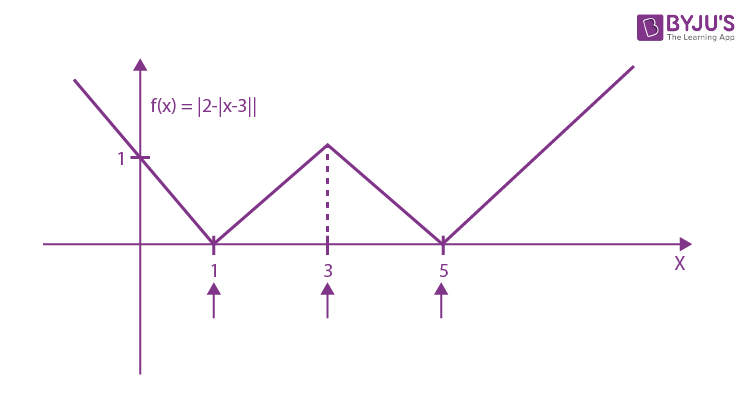Solution:

There will be three points 𝑥 = 1, 3, 5 at which f(x) is non-differentiable.

So f(f(1)) + f(f(3)) + f(f(5))

= f(0) + f(2) + f(0)

= 1+1+1

= 3

Question 25. Let A(1,0), B(6,2), C(3/2, 6) be the vertices of a triangle ABC. If P is a point inside the triangle ABC such that the triangle APC, APB and BPC have equal areas, then the length of the line the segment PQ, where Q is the point ( -7/6, -1/3), is

P is the centroid which is =

$$\begin{array}{l}\left ( \frac{1+6+\frac{3}{2}}{3} , \frac{0+2+6}{3}\right )\end{array}$$

P = (17/6, 8/3)

Q = (-7/6, -1/3)

PQ =

$$\begin{array}{l}\sqrt{4^{2}+3^{2}} = 5\end{array}$$